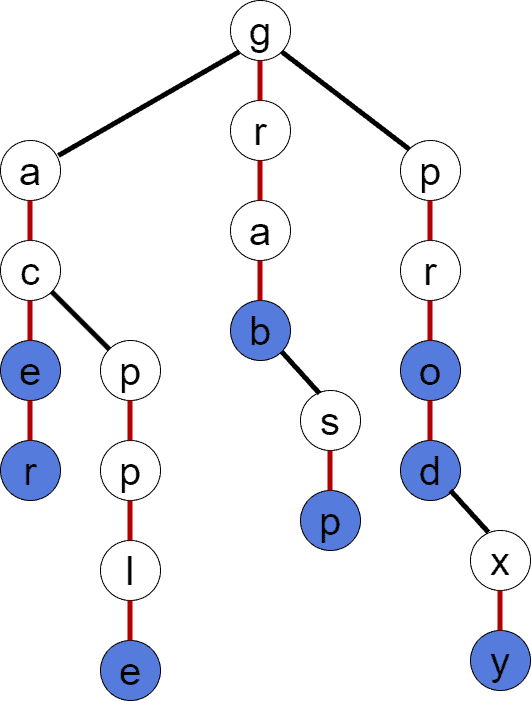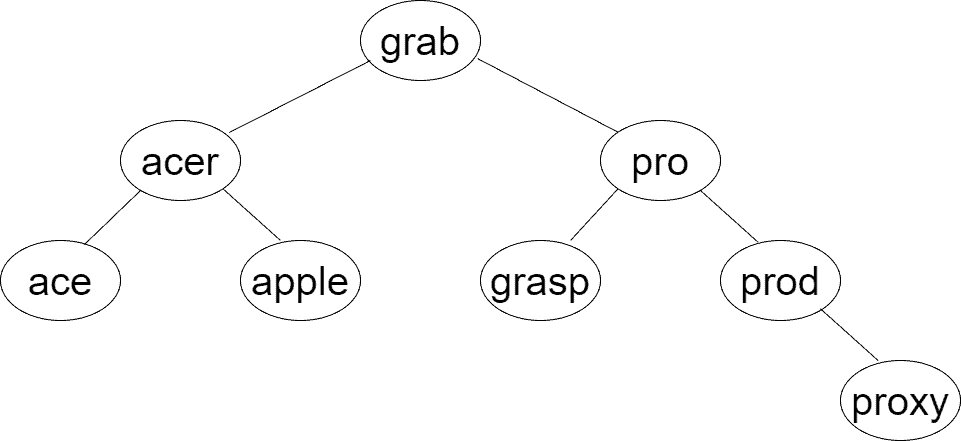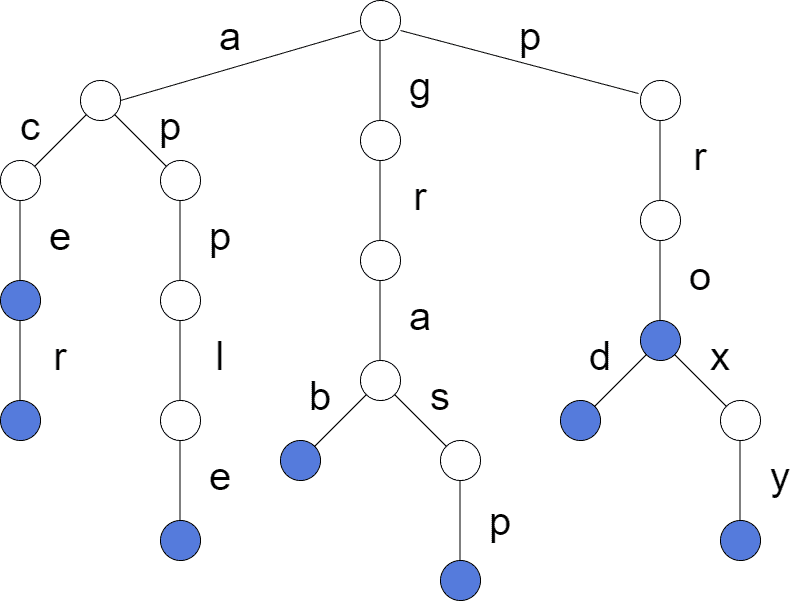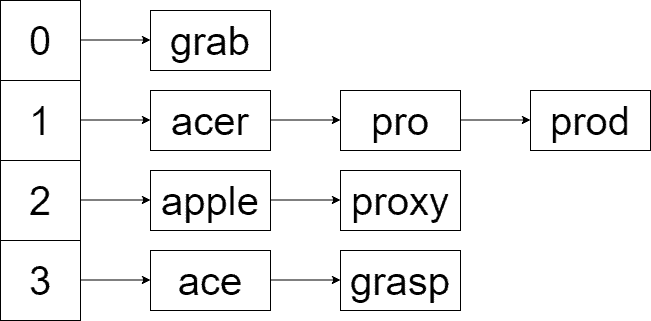## 1. Introduction

In this tutorial, we’ll cover the Ternary Search Tree (TST) data structure. It’s a very interesting data structure that can solve the problem of fast string lookup.

## 2. Initial Setup

Let’s assume we have a set of strings. Without losing generality, we’ll assume the strings inare lexicographically sorted. The lexicographical order will simplify our discussion. We’ll also treat the strings as 1-indexed sequences of symbols.

For our complexity estimations, let’s denote:

• The number of strings inby.
• The maximum string length inby.
• And the number of alphabet symbols used to construct strings by.

We’ll use the previously established sets and notations in the following sections of this tutorial.

## 3. An Overview of TST

TST has been employed as an efficient solution for frequent string lookups in a set of stringsIt offers the space efficiency of BST while providing the lightning-fast performance of Trie for searching strings.

TST is similar to Trie in terms of its structure – it splits string symbols and creates a tree out of them. Similarly to Trie and BST, TST consists of nodes, and each node contains:

•– a single symbol/occurrence in a string.
•– a reference to the left child node; the value in the left node is less than the value in the parent node.
•– a reference to the right child node; the value in the right node is greater than the value in the parent node.
•– a reference to the middle child node; the value in the middle node is the next symbol in a string which contains the value in the parent node followed by the value in the middle node.
•– a flag indicating whether the node is terminal, i.e., there’s a string inending in the node.

Each node in TST has up to 3 children, hence the data structure’s name. The figure next depicts a TST structure constructed out of the strings in. In this case, blue nodes are terminating ones. The terminating nodes mark the end of a word in. Red edges are middle children’s references. Seeing a red edge means at least one string incontainingfollowed by:Similarly to BST, the performance of TST is strictly dependent on the tree depthThe lookup performance of TST is, assuming TST is balanced. The estimation is derived from the fact that each symbol lookup – with a positive or negative result – may take up totime. Practically, the multiplier is much less than, as not all symbols are present at each level of the tree.

Overall space usage depends on the number of nodes in TST. The number of nodes, in turn, relies on the number of strings inthat share common prefixes. Thus, in the worst case with minimum prefix sharing, the space complexity is.

## 4. The TST Operations

In this section, we’ll go over the general TST operations. Note that there’s no string removal operation, as it’s not common for TST. The strings are generally added to the structure without removal afterward.

### 4.1. String Insertion

The insertion operation is a bit subtle. It needs to handle the following:

1. The creation of new tree nodes.
2. The child nodes initialization for the newly created and existing nodes.
3. Marking the nodes as terminal if the inserted word ends in an existing non-terminal node.

For simplicity, let’s see the implementation of an auxiliary node creation operation. We’ll assume there’s a data type TSTNode =:And we have a recursive version of the insertion operation next:The intuition behind insertion is that we need to insert the current symbol if we’re in a NULL node. The recursion then takes care of assigning the newly created node to the appropriate child of the parent node when the call returns. If we have a match at a node, then there’s no need to create a node for the current symbol, and we need to consider the next symbol of the string and follow the middle subtree. Additionally, if there’s a match and it’s the last symbol of the string, we mark the node as terminal. Contrarily, if there’s no match at a node, insertion continues in either the left or the right subtree with the current symbol.

### 4.2. Building TST

TST creation is actually a series of insertion operations. As in the case of BST, we have the worst-performing TST when inserting strings in sorted order.

There’re two cardinally different cases to consider when building TST:

1.is known beforehand, i.e., the offline mode. To have a well-balanced TST, consider inserting strings randomly. Another option is to sort the strings in, insert the middle string, and then continue insertions recursively in the left and right subsets of. Note that these approaches are directly derived from BST.
2.is dynamic, i.e., the online mode. In this case, we should use balancing techniques to keep a good TST performance.

Of course, we can always add balancing logic to our TST to guarantee performance.

Let’s write the pseudocode for the build operation in case of the offline mode. We’ll employ the version of random string insertion. To achieve randomness, we first shuffle the strings inand then insert them in order:### 4.3. String Lookup

We have the pseudocode for a recursive string lookup in TST shown below:freestar.config.enabled_slots.push({ placementName: "baeldung_incontent_1", slotId: "baeldung_incontent_1" });



Initially, the function is invoked on the TST’s root node to look up the string’s first symbol: INSERT(). So, the first condition indicates the case when the string isn’t found. It’s reached when there’re still symbols in the string to search for, but there’s no way to go in the TST.

The following two conditions are for the cases when there’s no match in the current node, and we need to continue the search in either the left or the right subtree. Finally, the remaining part of the function handles the match and indicates if the search has finished or if we need to continue the search in the middle subtree.

## 5. Applications

There’re a lot of practical applications of TST. It may be used anywhere Trie is used. Thus, let’s see some typical applications of TST:

• Searching for words in a dictionary.
• Finding all strings that have a common prefix.
• Suggestions for word autocomplete when typing a text.
• Suggestions for query autocomplete when typing a query in a search engine.
• Finding the lexicographically next/previous string.

## 6. Alternative Data Structures

This section will discuss alternative data structures used in string lookup. Indeed, there are several of them, and each has its own peculiarities.

### 6.1. BST

In the following figure, we can see a possible balanced BST constructed from:A balanced BST offersperformance during a general lookup. However, when keys are of the string type, there’s an additional complexity multiplier. The overall complexity for a string lookup isbecause every single key comparison is anstring comparison.

Consider the lookup of. After comparing the first characters ofand, the search goes to the right branch. Now,is fully compared tosymbol by symbol until there’s no match, and we need to go right.

The same brute-force comparison is made when comparingto. And finally, we findin the rightmost node of the tree. The evident drawback of BST for searching strings is redundant comparisons of common prefixes. We need a faster way to skip the current key and move forward.

Furthermore, the space usage of BST is.

### 6.2. Trie

Trie is the best data structure for string lookup in terms of speedBut, it uses a lot of redundant space and isn’t always applicable. Below, we can see the Trie for; the terminating nodes are colored in blue. Note that for image simplicity, we haven’t visualized the redundant references kept at each node:The specifics of the Trie structure allow us to look up a string intime. Tries are fast because each node keeps the (random-access) array of references to indicate which symbols branch out from a node. So, it’s possible to tell intime if the search needs to continue for any symbol of the search string at any node in Trie. Since there aresymbols in a string, the lookup works in.

However, the space requirement is huge – each node takesspace. For example, if our alphabet consists of uppercase and lowercase English letters, each node keeps 52 references. So, most of the time, we have lots of redundant references. Furthermore, the overall space usage, as in the case of TST, depends on the number of nodes in Trie. In the worst case with minimum prefix sharing, the space complexity goes up to.

### 6.3. Hash Table

Hash tables are known as the best general lookup data structure. Below is the hash table for, with 4 slots and the hash function:Hash tables offer amortizedlookup by generally assuming the use of anhash function. When storing strings, there’s usually amultiplier for computing the hash value. Similarly to the BST case, there’s amultiplier for comparing a constant number of strings in the slot to find the searched string.

So, the lookup runs in amortizedtime. Moreover, space usage is, where, in such a case, is the number of slots in the hash table.

Additionally, hash tables keep the data unordered. Contrarily, the rest of the discussed data structures are ordered. Thus, a hash table isn’t an option for applications where ordering matters, despite the good practical performance.

## 7. Conclusion

In this tutorial, we studied the TST data structure. Initially, the main concepts regarding TST were presented. Thus, we discussed the TST operations and provided pseudocodes for them. Then, we enumerated practical applications of this data structure. Finally, we discussed some alternative data structures for string lookup.